Next: Order Up: Implementation Previous: Optimization with function and   Contents

Return code

The procedure will return an integer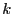that will be identical to the code returned by the procedure
•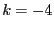: one of the equation in the system has not a type 0, -1, -2, 2, 10 or 1 (i.e. it's not an equation or an optimum function, neither inequality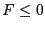or an inequality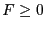)
•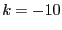: there is no optimum function i.e. no equation has type 2 or -2 or 10
•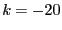: there is more than one optimum function i.e. more than one equation has type 2 or -2 or 10
•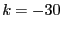: the last function is not the one to be minimized or maximized